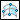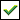Surfacing > Creo Reverse Engineering (Restyle) > Creating Polynomial Surfaces in Restyle > To Create a Rectangular Surface Using the End Points of a Cross
To Create a Rectangular Surface Using the End Points of a Cross
1. Click Restyle > Polynomial Surface >Midpoints. The 4 Points - Cross mathematical properties dialog box opens.
2. Holding down the CTRL key, select four points. A rectangular surface is created where each boundary has one of the selected points roughly at the boundary midpoint.
3. Define the mathematical properties of the surface:
a. Select a surface type.
b. In the Degree box, type or select a value from 1 to 15.
c. In the Number of segments box, type or select a value from 2 to 200.
d. Click OK to close the 4 Points - Cross dialog box.
4. ClickOK.